# The quality control manager at a compact fluorescent light bulb (CFL) factory needs to determine whether...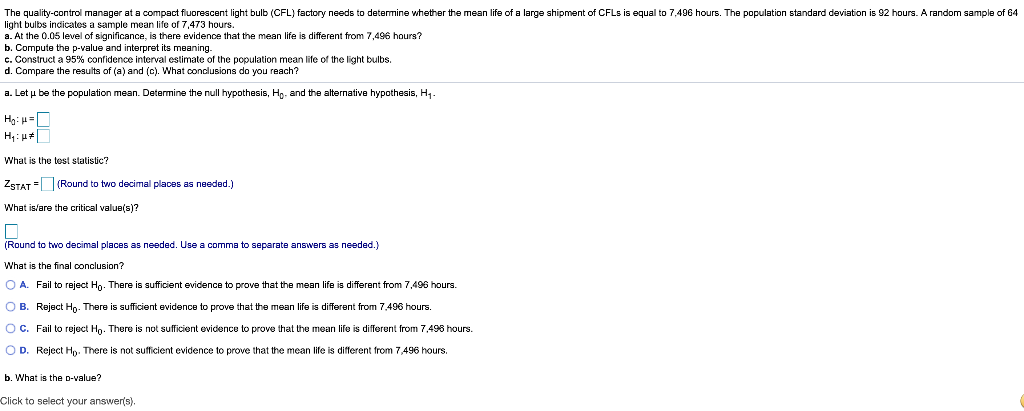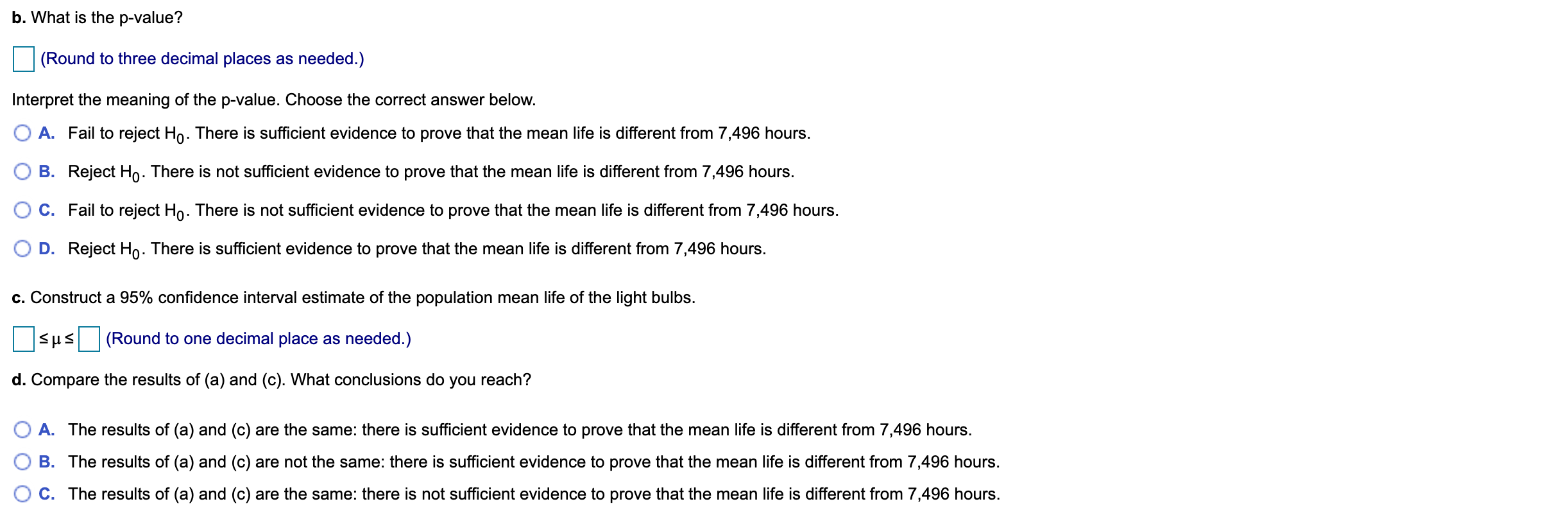The quality control manager at a compact fluorescent light bulb (CFL) factory needs to determine whether the mean life of a large shipment of CFLs is equal to 7.496 hours. The population standard deviation is 92 hours. A random sample of 64 light bulbs indicatos a sample mean life of 7,473 hours. a. At the 0.05 level of significance, is there evidence that the mean life is different from 7,496 hours? b. Compute the p-value and interpret its meaning. c. Construct a 95% confidence interval estimate of the population mean life of the light bulbs d. Compare the results of (a) and (c). What conclusions do you reach? a. Let y be the population mean. Determine the null hypothesis, Ho, and the alternative hypothesis, H, HON= H: * What is the best statistic? ZSTAT (Round to two decimal places as needed.) What is/are the critical value(s)? (Round to two decimal places as needed. Use a comma to separate answers as needed.) What is the final conclusion? O A. Fail to reject Ho. There is sufficient evidence to prove that the mean life is different from 7,496 hours. OB. Reject Hp. There is sufficient evidence to prove that the mean life is different from 7.496 hours. O C . Fail to reject Ho. There is not sufficient evidence to prove that the mean life is different from 7.498 hours. OD. Reject Ho. There is not sufficient evidence to prove that the mean life is different from 7,496 hours. b. What is the D-value? Click to select your answer(s)
b. What is the p-value? (Round to three decimal places as needed.) Interpret the meaning of the p-value. Choose the correct answer below. O A. Fail to reject Ho. There is sufficient evidence to prove that the mean life is different from 7,496 hours. OB. Reject Ho. There is not sufficient evidence to prove that the mean life is different from 7,496 hours. O C. Fail to reject Ho. There is not sufficient evidence to prove that the mean life is different from 7,496 hours. OD. Reject Ho. There is sufficient evidence to prove that the mean life is different from 7,496 hours. c. Construct a 95% confidence interval estimate of the population mean life of the light bulbs. sus (Round to one decimal place as needed.) d. Compare the results of (a) and (c). What conclusions do you reach? O A. The results of (a) and (c) are the same: there is sufficient evidence to prove that the mean life is different from 7,496 hours. OB. The results of (a) and (c) are not the same: there is sufficient evidence to prove that the mean life is different from 7,496 hours. O C. The results of (a) and (c) are the same: there is not sufficient evidence to prove that the mean life is different from 7,496 hours.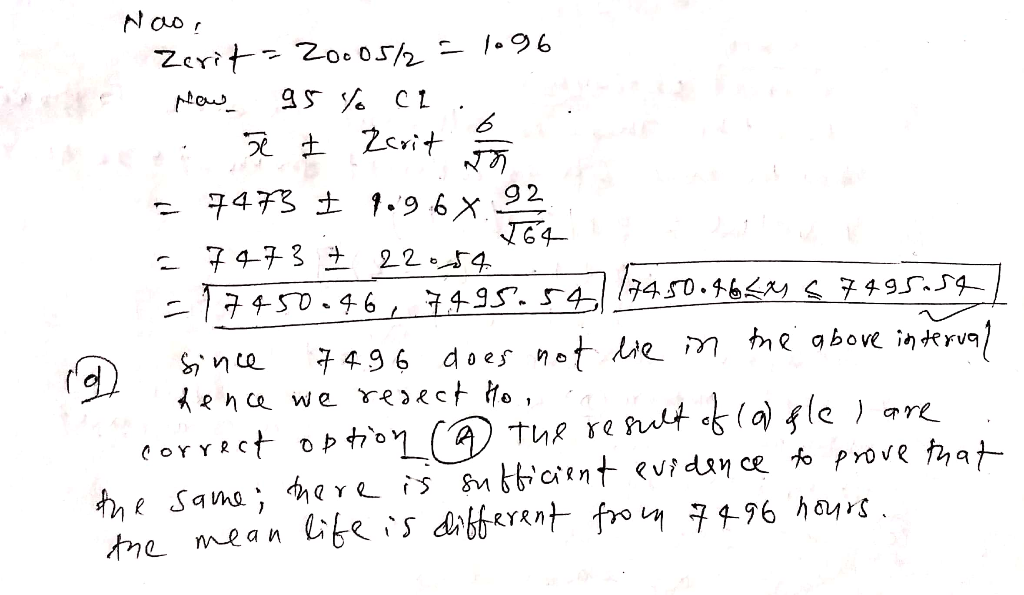##### Add Answer to: The quality control manager at a compact fluorescent light bulb (CFL) factory needs to determine whether...
Similar Homework Help Questions
• ### The quality-control manager at a compact fluorescent light bulb (CFL) factory needs to determine whether the...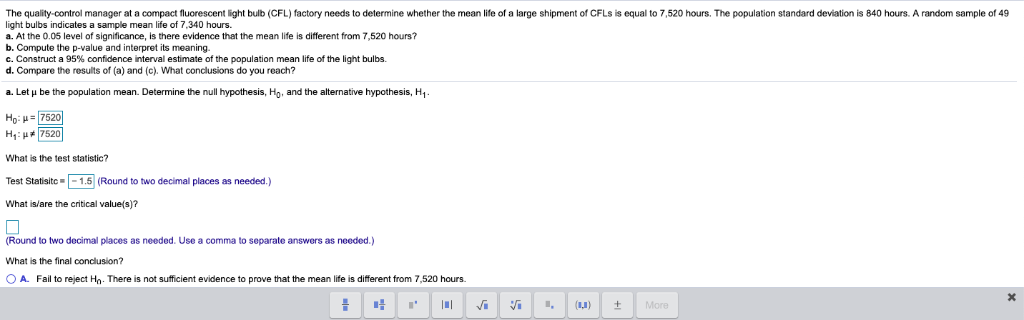The quality-control manager at a compact fluorescent light bulb (CFL) factory needs to determine whether the mean life of a large shipment of CFLs is equal to 7,520 hours. The population standard deviation is 840 hours. A random sample of 49 light bulbs indicates a sample mean life of 7,340 hours. a. At the 0.05 level of significance, is there evidence that the mean life is different from 7,520 hours? b. Compute the p-value and interpret its meaning. c. Construct...

• ### I will be sure to rate well, Thanks! The quality-control manager at a compact fluorescent light...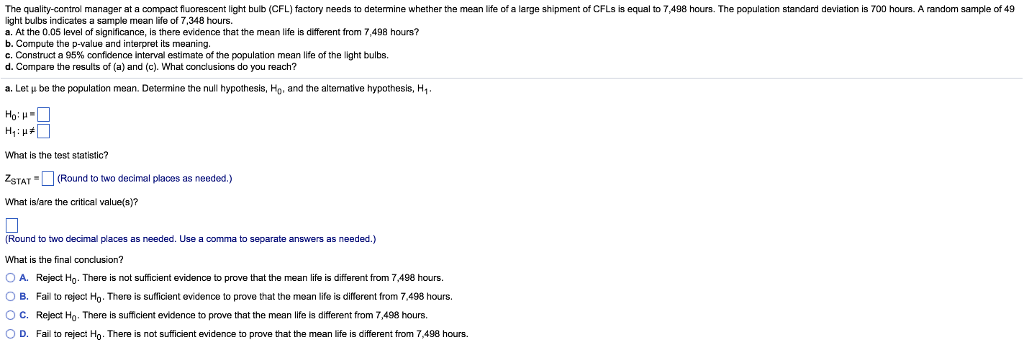I will be sure to rate well, Thanks! The quality-control manager at a compact fluorescent light bulb (CFL) factory needs to determine whether the mean life of a large shipment of CFLs is equal to 7,498 hours. The population standard deviation is 700 hours. A random sample of 49 light bulbs indicates a sample mean life of 7,348 hours. a. At the 0.05 level of significance, is there evidence that the mean life is different from 7,498 hourst b. Compute...

• ### The quality control manager at a light bulb factory needs to determine whether the mean life...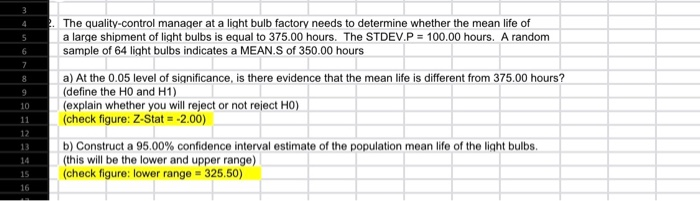The quality control manager at a light bulb factory needs to determine whether the mean life of a large shipment of light bulbs is equal to 375.00 hours. The STDEV.P = 100.00 hours. A random sample of 64 light bulbs indicates a MEAN.S of 350.00 hours a) At the 0.05 level of significance, is there evidence that the mean life is different from 375.00 hours? (define the Ho and H1) (explain whether you will reject or not reject HO) (check...

• ### A light bulb manufacturer guarantees that the mean life of a certain type of light bulb...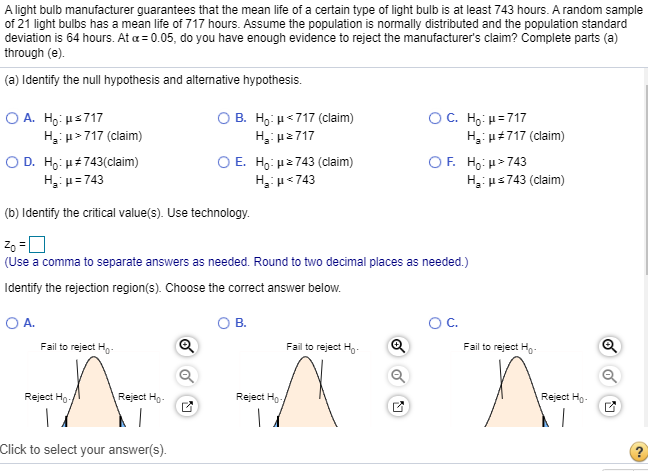A light bulb manufacturer guarantees that the mean life of a certain type of light bulb is at least 743 hours. A random sample of 21 light bulbs has a mean life of 717 hours. Assume the population is normally distributed and the population standard deviation is 64 hours. At a = 0.05, do you have enough evidence to reject the manufacturer's claim? Complete parts (a) through (e) (a) Identify the null hypothesis and alternative hypothesis. O A. Ho: \$717...

• ### The quality-control manager at a light bulb factory needs to determine wheter the mean life of a...

The quality-control manager at a light bulb factory needs to determine wheter the mean life of a population of light bulbs is equal to 375 hours. A random sample of 64light bulbs indicates a sample mean of 350 hours and a standard deviation of 100 hours. At a 5% level of significance, is there evidence to conclude that the mean lifeis different from 375 hours?

• ### A bottled water distributor wants to determine whether the mean amount of water contained in 1-gallon bottles purchased from a nationally known water bottling company is actually 1 gallon. You know f...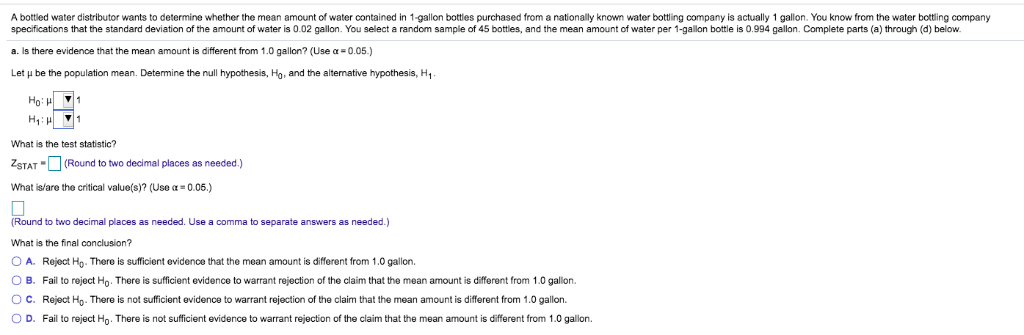A bottled water distributor wants to determine whether the mean amount of water contained in 1-gallon bottles purchased from a nationally known water bottling company is actually 1 gallon. You know from the water bottling company specifications that the standard deviation of the amount of water is 0.02 gallon. You select a random sample of 45 bottles, and the mean amount of water per 1-gallon bottle is 0.994 gallon. Complete parts (a) through (d) below. a. Is there evidence that...

• ### A light bulb manufacturer guarantees that the mean life of a certain type of light bulb...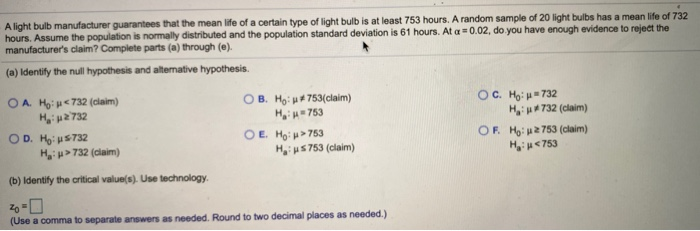A light bulb manufacturer guarantees that the mean life of a certain type of light bulb is at least 753 hours. A random sample of 20 light bulbs has a mean life of 732 hours. Assume the population is normally distributed and the population standard deviation is 61 hours. At a =0.02, do you have enough evidence to reject the manufacturer's claim? Complete parts (a) through (o). (a) Identify the null hypothesis and alternative hypothesis. O A. Ho: < 732...

• ### A light bulb manufacturer guarantees that the mean life of a certain type of light bulb...

A light bulb manufacturer guarantees that the mean life of a certain type of light bulb is at least 753 hours. A random sample of 20 light bulbs has a mean life of 732 hours. Assume the population is normally distributed and the population standard deviation is 61 hours. At α=0.02​, do you have enough evidence to reject the​ manufacturer's claim? Complete parts​ (a) through​ (e). ​(a) Identify the null hypothesis and alternative hypothesis. A. H0​: μ<732 ​(claim) Ha​: μ≥732...

• ### A random sample of 100 observations from a population with standard deviation 76 yielded a sample...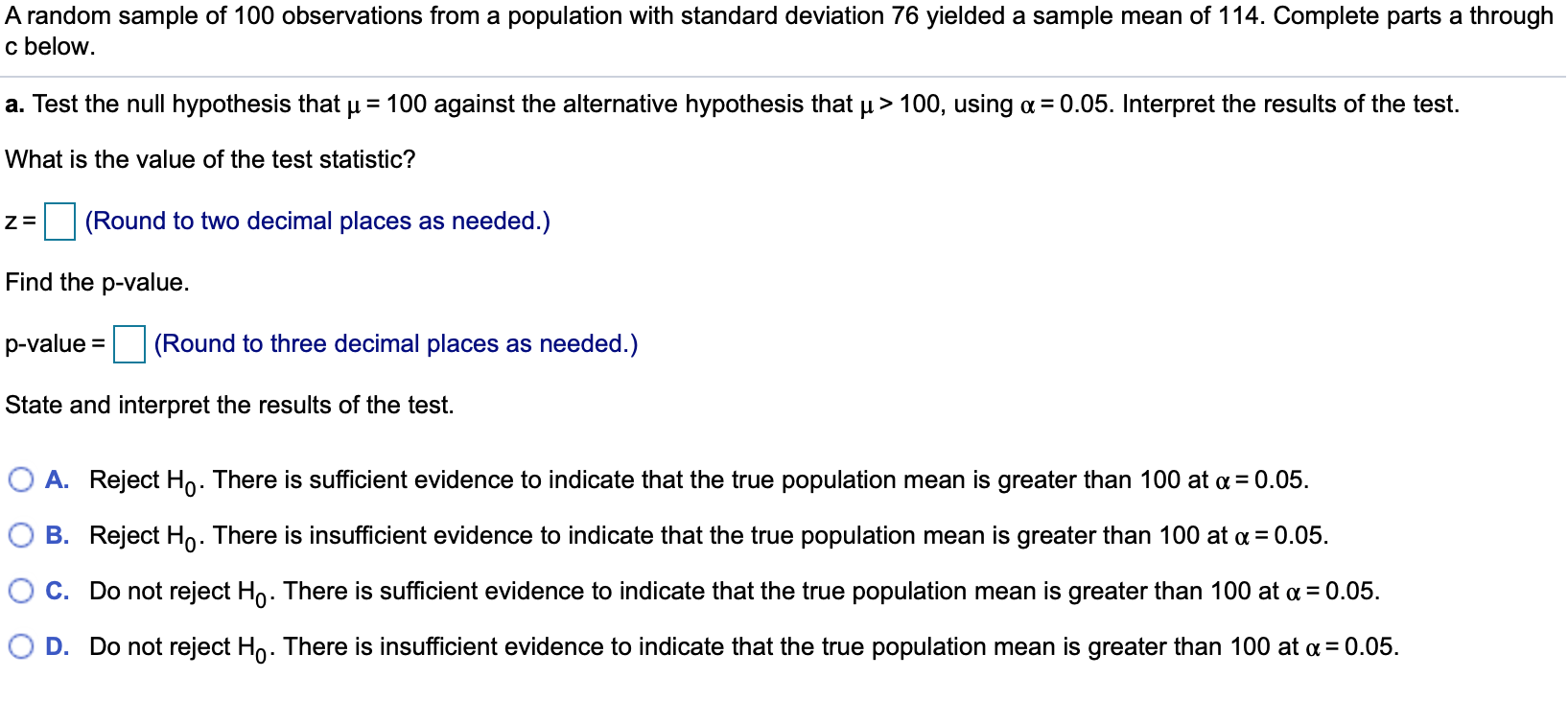A random sample of 100 observations from a population with standard deviation 76 yielded a sample mean of 114. Complete parts a through c below. a. Test the null hypothesis that u = 100 against the alternative hypothesis that u > 100, using a = 0.05. Interpret the results of the test. What is the value of the test statistic? und to two decimal places as needed.) Find the p-value. p-value = (Round to three decimal places as needed.) State...

• ### A nutritionist claims that the mean tuna consumption by a person is 3.5 pounds per year....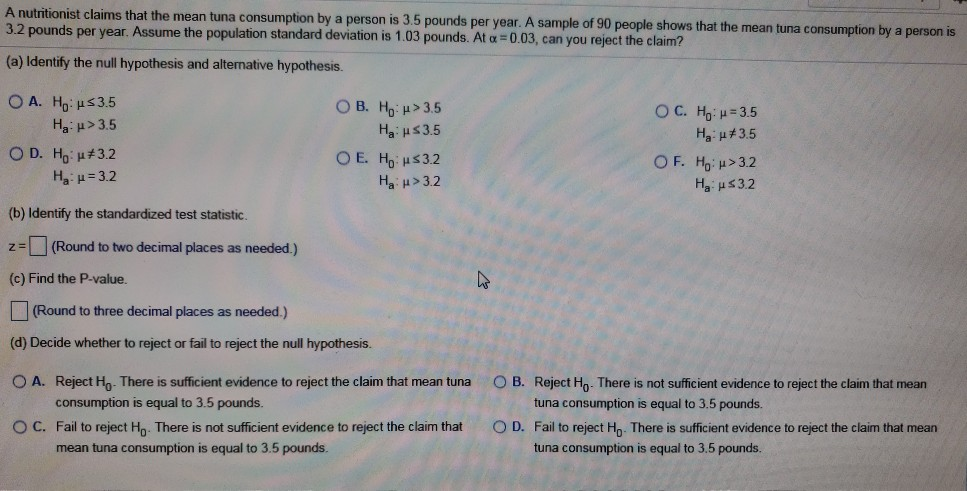A nutritionist claims that the mean tuna consumption by a person is 3.5 pounds per year. A sample of 90 people shows that the mean tuna consumption by a person is 3.2 pounds per year. Assume the population standard deviation is 1.03 pounds. At c =0.03, can you reject the claim? (a) Identify the null hypothesis and alternative hypothesis. O A. Ho: 53.5 Ha: > 3.5 OD. Hou#3.2 Hau = 3.2 O B. Ho: > 3.5 Ha \$3.5 O E....

Free Homework App